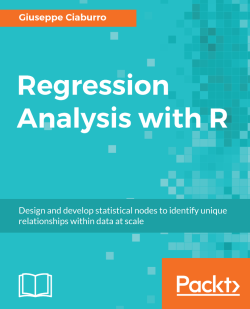# Regression Analysis with R5 (2 reviews total)
By Giuseppe Ciaburro
• \$33.99 eBook
• \$41.99 Print + eBook
• \$12.99 eBook + Subscription
What do you get with a Packt Subscription?

• Constantly updated with 100+ new titles each month
• Breadth and depth in over 1,000+ technologies

Regression analysis is a statistical process which enables prediction of relationships between variables. The predictions are based on the casual effect of one variable upon another. Regression techniques for modeling and analyzing are employed on large set of data in order to reveal hidden relationship among the variables.

This book will give you a rundown explaining what regression analysis is, explaining you the process from scratch. The first few chapters give an understanding of what the different types of learning are – supervised and unsupervised, how these learnings differ from each other. We then move to covering the supervised learning in details covering the various aspects of regression analysis. The outline of chapters are arranged in a way that gives a feel of all the steps covered in a data science process – loading the training dataset, handling missing values, EDA on the dataset, transformations and feature engineering, model building, assessing the model fitting and performance, and finally making predictions on unseen datasets. Each chapter starts with explaining the theoretical concepts and once the reader gets comfortable with the theory, we move to the practical examples to support the understanding. The practical examples are illustrated using R code including the different packages in R such as R Stats, Caret and so on. Each chapter is a mix of theory and practical examples.

By the end of this book you will know all the concepts and pain-points related to regression analysis, and you will be able to implement your learning in your projects.

Publication date:
January 2018
Publisher
Packt
Pages
422
ISBN
9781788627306

## Chapter 1. Getting Started with Regression

Regression analysis is the starting point in data science. This is because regression models represent the most well-understood models in numerical simulation. Once we experience the workings of regression models, we will be able to understand all other machine learning algorithms. Regression models are easily interpretable as they are based on solid mathematical bases (such as matrix algebra for example). We will see in the following sections that linear regression allows us to derive a mathematical formula representative of the corresponding model. Perhaps this is why such techniques are extremely easy to understand.

Regression analysis is a statistical process done to study the relationship between a set of independent variables (explanatory variables) and the dependent variable (response variable). Through this technique, it will be possible to understand how the value of the response variable changes when the explanatory variable is varied.

Consider some data that is collected about a group of students, on: number of study hours per day, attendance at school, and scores on the final exam obtained. Through regression techniques, we can quantify the average increase in the final exam score when we add one more hour of study. Lower attendance in school (decreasing the student's experience) lowers the scores in the final exam.

A regression analysis can have two objectives:

• Explanatory analysis: To understand and weigh the effects of the independent variable on the dependent variable according to a particular theoretical model
• Predictive analysis: To locate a linear combination of the independent variable to predict the value assumed by the dependent variable optimally

In this chapter, we will be introduced to the basic concepts of regression analysis, and then we'll take a tour of the different types of statistical processes. In addition to this, we will also introduce the R language and cover the basics of the R programming environment. Finally we will explore the essential tools that R provides for understanding the amazing world of regression.

We will cover the following topics:

• The origin of regression
• Types of algorithms
• How to quickly set up R for data science
• R packages used throughout the book

At the end of this chapter, we will provide you with a working environment that is able to run all the examples contained in the following chapters. You will also get a clear idea about why regression analysis is not just an underrated technique taken from statistics, but a powerful and effective data science algorithm.

## Going back to the origin of regression

The word regression sounds like go back in some ways. If you've been trying to quit smoking but then yield to the desire to have a cigarette again, you are experiencing an episode of regression. The term regression has appeared in early writings since the late 1300s, and is derived from the Latin term regressus, which means a return. It is used in different fields with different meanings, but in any case, it is always referred to as the action of regressing.

In philosophy, regression is used to indicate the inverse logical procedure with respect to that of the normal apodictic (apodixis), in which we proceed from the general to the particular. Whereas in reality, in regression we go back from the particular to the general, from the effect to the cause, from the conditioned to the condition. In this way, we can draw completely general conclusions from a particular case. This is the first form of generalization that, as we will see later, represents a crucial part of a statistical analysis.

For example, there is marine regression, which is a geological process that occurs as a result of areas of submerged seafloor being exposed more than the sea level. And there is the opposite event, marine transgression; it occurs when flooding from the sea submerges land that was previously exposed.

The following image shows the results of marine regression on the Aral lake.  This lake lies between Kazakhstan and Uzbekistan and has been steadily shrinking since the 1960s, after the rivers that fed it were diverted by Soviet irrigation projects. By 1997, it had declined to ten percent of its original size:However, in statistics, regression is related to the study of the relationship between the explanatory variables and the response variable. Don't worry! This is the topic we will discuss in this book.

In statistics, the term regression has an ancient origin and a very special meaning. The man who coined this term was a certain Francis Galton, geneticist, who in 1889 published an article in which he demonstrated how every characteristic of an individual is inherited by their offspring, but on an average to a lesser degree. For example, children with tall parents are also tall, but on an average their height will be comparatively less than that of their parents. This phenomenon, also graphically described, is called regression. Since then, this term has lived on to define statistical techniques that analyze relationships between two or more variables.

The following table shows a short summary of the data used by Galton for his study:

 Family Father Mother Gender Height Kids `1` `78.5` `67` `M` `73.2` `4` `1` `78.5` `67` `F` `69.2` `4` `1` `78.5` `67` `F` `69` `4` `1` `78.5` `67` `F` `69` `4` `2` `75.5` `66.5` `M` `73.5` `4` `2` `75.5` `66.5` `M` `72.5` `4` `2` `75.5` `66.5` `F` `65.5` `4` `2` `75.5` `66.5` `F` `65.5` `4` `3` `75` `64` `M` `71` `2` `3` `75` `64` `F` `68` `2` `4` `75` `64` `M` `70.5` `5` `4` `75` `64` `M` `68.5` `5` `4` `75` `64` `F` `67` `5` `4` `75` `64` `F` `64.5` `5` `4` `75` `64` `F` `63` `5` `5` `75` `58.5` `M` `72` `6` `5` `75` `58.5` `M` `69` `6` `5` `75` `58.5` `M` `68` `6` `5` `75` `58.5` `F` `66.5` `6` `5` `75` `58.5` `F` `62.5` `6` `5` `75` `58.5` `F` `62.5` `6`

Galton depicted in a chart the average height of the parents and that of the children, noting what he called regression in the data.

The following figure shows this data and a regression line that confirms his thesis: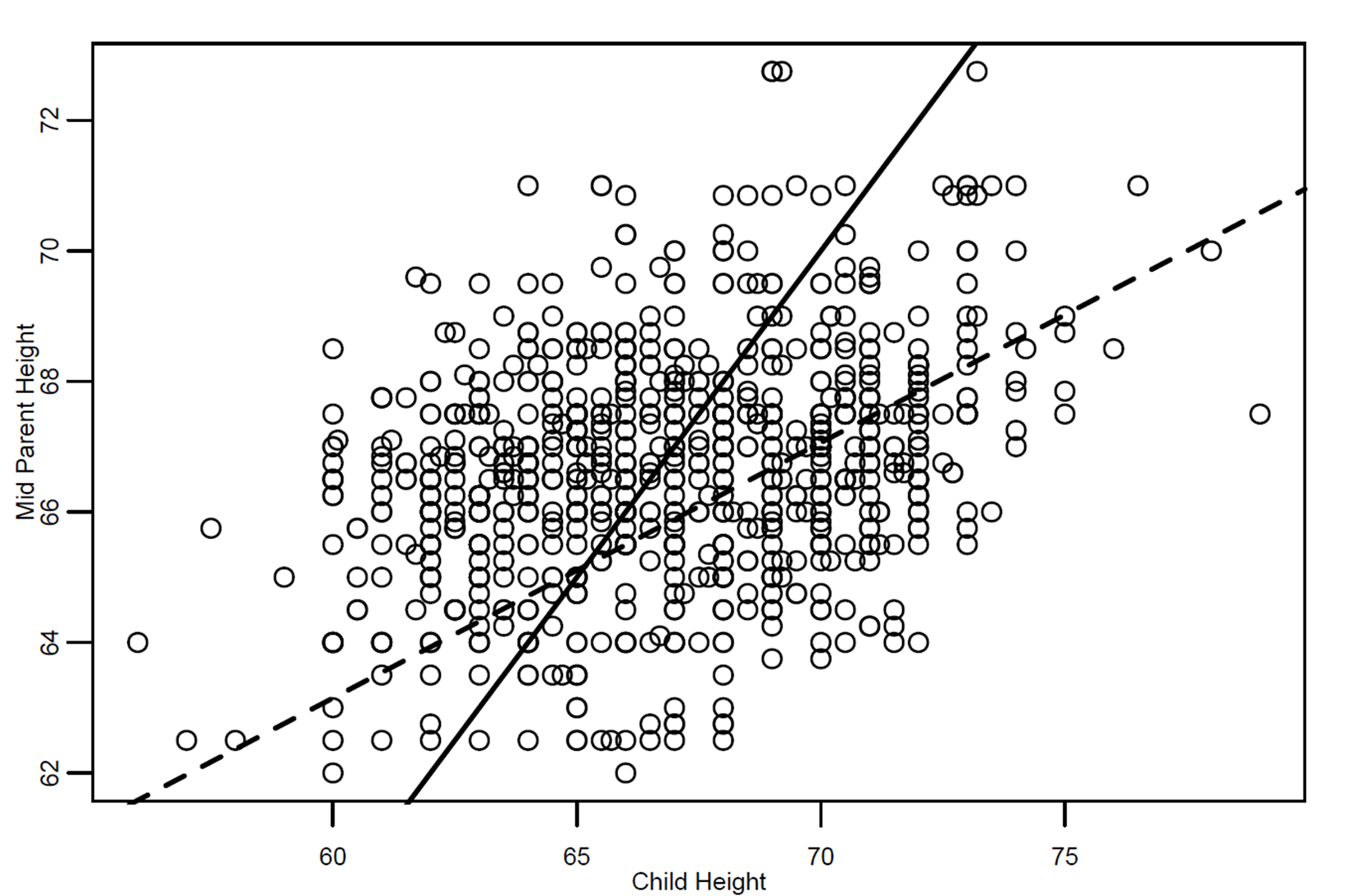The figure shows a scatter plot of data with two lines. The darker straight line represents the equality line, that is, the average height of the parents is equal to that of the children. The straighter dotted line, on the other hand, represents the linear regression line (defined by Galton) showing a regression in the height of the children relative to that of the parents. In fact, that line has a slope less than that of the continuous line.

Indeed, the children with tall parents are also tall, but on an average they are not as tall as their parents (the regression line is less than the equality line to the right of the figure). On the contrary, children with short parents are also short, but on an average, they are taller than their parents (the regression line is greater than the equality line to the left of the figure). Galton noted that, in both cases, the height of the children approached the average of the group. In Galton's words, this corresponded to the concept of regression towards mediocrity, hence the name of the statistical analysis technique.

The Galton universal regression law was confirmed by Karl Pearson (Galton's pupil), who collected more than a thousand heights of members of family groups. He found that the average height of the children from a group of short parents was greater than the average height of their fathers' group, and that the average height of the children from a group of tall fathers was lower than the average height of their parents' group. That is, the heights of the tall and short children were regressing toward the average height of all people. This is exactly what we have learned from the observation of the previous graph.

## Regression in the real world

In general, statistics—and more specifically, regression—is a math discipline. Its purpose is to obtain information from data about knowledge, decisions, control, and the forecasting of events and phenomena. Unfortunately, statistical culture, and in particular statistical reasoning, are scarce and uncommon. This is due to the institutions that have included the study of this discipline in their programs and study plans inadequately. Often, inadequate learning methods are adopted since this is a rather complex and not very popular topic (as is the case with mathematics in general).

The difficulties faced by students are often due to outdated teaching methods that are not in tune with our modern needs. In this book, we will learn how to deal with such topics with a modern approach, based on practical examples. In this way, all the topics will seem simple and within our reach.

Yet regression, given its cross-disciplinary characteristics, has numerous and varied areas of application, from psychology to agrarianism, and from economics to medicine and business management, just to name a few.

The purpose of regression as a statistical tool are of two types, synthesize and generalize, as shown in the following figure: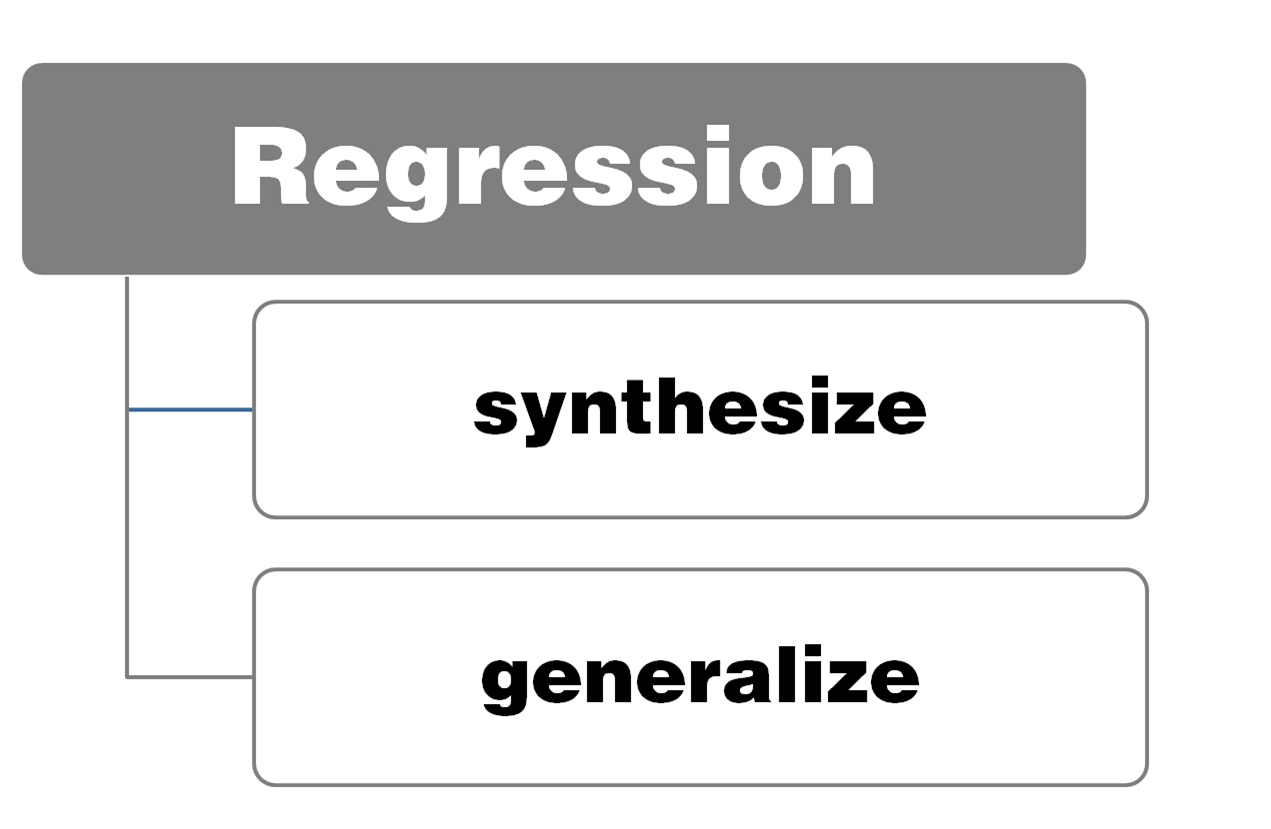synthesize means predisposing collected data into a form (tables, graphs, or numerical summaries), which allows you to better understand the phenomena on which the detection was performed. The synthesis is met by the need to simplify, which in turn results from the limited ability of the human mind to handle articulated, complex, or multidimensional information. In this way, we can use techniques that allow for a global study of a large number of quantitative and qualitative information to highlight features, ties, differences, or associations between detected variables.

The second purpose (generalize) is to extend the result of an analysis performed on data of a limited group of statistical units (sample) to the entire population group (population).

The contribution of regression is not limited to the data analysis phase. It's true that added value is expressed in the formulation of research hypotheses, argumentation of theses, adoption of appropriate solutions and methodologies, choices of methods of detection, formulation of the sample, and the procedure of extending the results to the reference universes.

Keeping these phases under control means producing reliable and economically useful results, and mastering descriptive statistics and data analysis as well as inferential ones. In this regard, we recall that the descriptive statistics are concerned with describing the experimental data with few significant numbers or graphs. Therefore, they photographs a given situation and summarizes its salient characteristics. The inferential statistics use statistical data, also appropriately summarized by the descriptive statistics, to make probabilistic forecasts on future or otherwise uncertain situations.

People, families, businesses, public administrations, mayors, ministers, and researchers constantly make decisions. For most of them, the outcome is uncertain, in the sense that it is not known exactly what will result, although the expectation is that they will achieve the (positive) effects they are hoping for. Decisions would be better and the effects expected closer to those desired if they were made on the basis of relevant data in a decision-making context. Here are some applications of regression in the real world:

• A student who graduates this year must choose the faculty and university degree course on which he/she will enroll. Perhaps he/she has already gained a vocation for his future profession, or studies have confirmed his/her predisposition for a particular discipline. Maybe a well-established family tradition advises him/her to follow the parent's profession. In these cases, the uncertainty of choice will be greatly reduced. However, if the student does not have genuine vocations or is not geared particularly to specific choices, he or she may want to know something about the professional outcomes of the graduates. In this regard, some statistical study on graduate data from previous years may help him/her make the decision.
• A distribution company, such as a supermarket chain, wants to open a new sales outlet in a big city and must choose the best location. It will use and analyze numerous statistical data on the density of the population in different neighborhoods, the presence of young families, the presence of children under the age of six (if it is interested in selling to this category of consumers), and the presence of schools, offices, other supermarkets, and retail outlets.
• Another company wants to invest its profits. It must make a portfolio choice. It has to decide whether to invest in government bonds, national shares, foreign securities, funds, or real estate. To make this choice, it will first conduct an analysis of the returns and risks of different investment alternatives based on statistical data.
• National governments are often called upon to make choices and decisions. To do this, they have statistical production equipment. They have population data and forecasts about population evolution over the coming years, which will calibrate their interventions. A strong decline in birth rates will, for example, recommend school consolidation policies; the emergence of children from the non-community component will signal the need for reviewing multi-ethnic programs and, more generally, school integration policies. On the other hand, statistical data on the presence of national products in foreign markets will suggest the need to export support actions or interventions to promote innovation and business competitiveness.

In the examples we have seen so far, the usefulness of statistical techniques, and particularly of regression in the most diverse working situations, is clear. It is therefore clear how much more information and data companies are required to have to ensure the rationality of decisions and economic behaviors by those who direct them.

## Understanding regression concepts

Regression is an inductive learning task that has been widely studied and is widely used in practical applications. Unlike classification processes, where you are trying to predict discrete class labels, regression models predict numeric values.

From a set of data, we can find a model that describes it by the use of the regression algorithms. For example, we can identify a correspondence between input variables and output variables of a given system. One way to do this is to postulate the existence of some kind of mechanism for the parametric generation of data; this, however, does not contain the exact values of the parameters. This process typically makes reference to statistical techniques.

The extraction of general laws from a set of observed data is called induction, as opposed to deduction in which we start from general laws and try to predict the value of a set of variables. Induction is the fundamental mechanism underlying the scientific method in which we want to derive general laws (typically described in mathematical terms) starting from the observation of phenomena. In the following figure, we can see Peirce's triangle, which represents a scheme of relationships between reasoning patterns: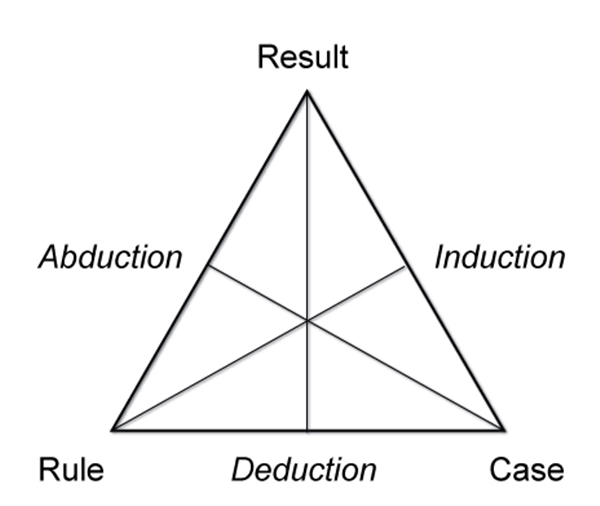The observation of the phenomena includes the measurement of a set of variables, and therefore the acquisition of data that describes the observed phenomena. Then, the resulting model can be used to make predictions on additional data. The overall process in which, starting from a set of observations, we aim to make predictions on new situations, is called inference.

Therefore, inductive learning starts with observations arising from the surrounding environment that, hopefully, are also valid for not-yet-observed cases.

We have already anticipated the stages of the inference process; now let's analyze them in detail through the workflow setting. When developing an application that uses regression algorithms, we will follow a procedure characterized by the following steps:

1. Collect the data: Everything starts with the data—no doubt about it—but one might wonder where so much data comes from. In practice, data is collected through lengthy procedures that may, for example, be derived from measurement campaigns or face-to-face interviews. In all cases, data is collected in a database so that it can then be analyzed to obtain knowledge.

### Note

If we do not have specific requirements, and to save time and effort, we can use publicly available data. In this regard, a large collection of data is available at the `UCI Machine Learning Repository`, at the following link: http://archive.ics.uci.edu/ml.

The following figure shows the regression process workflow: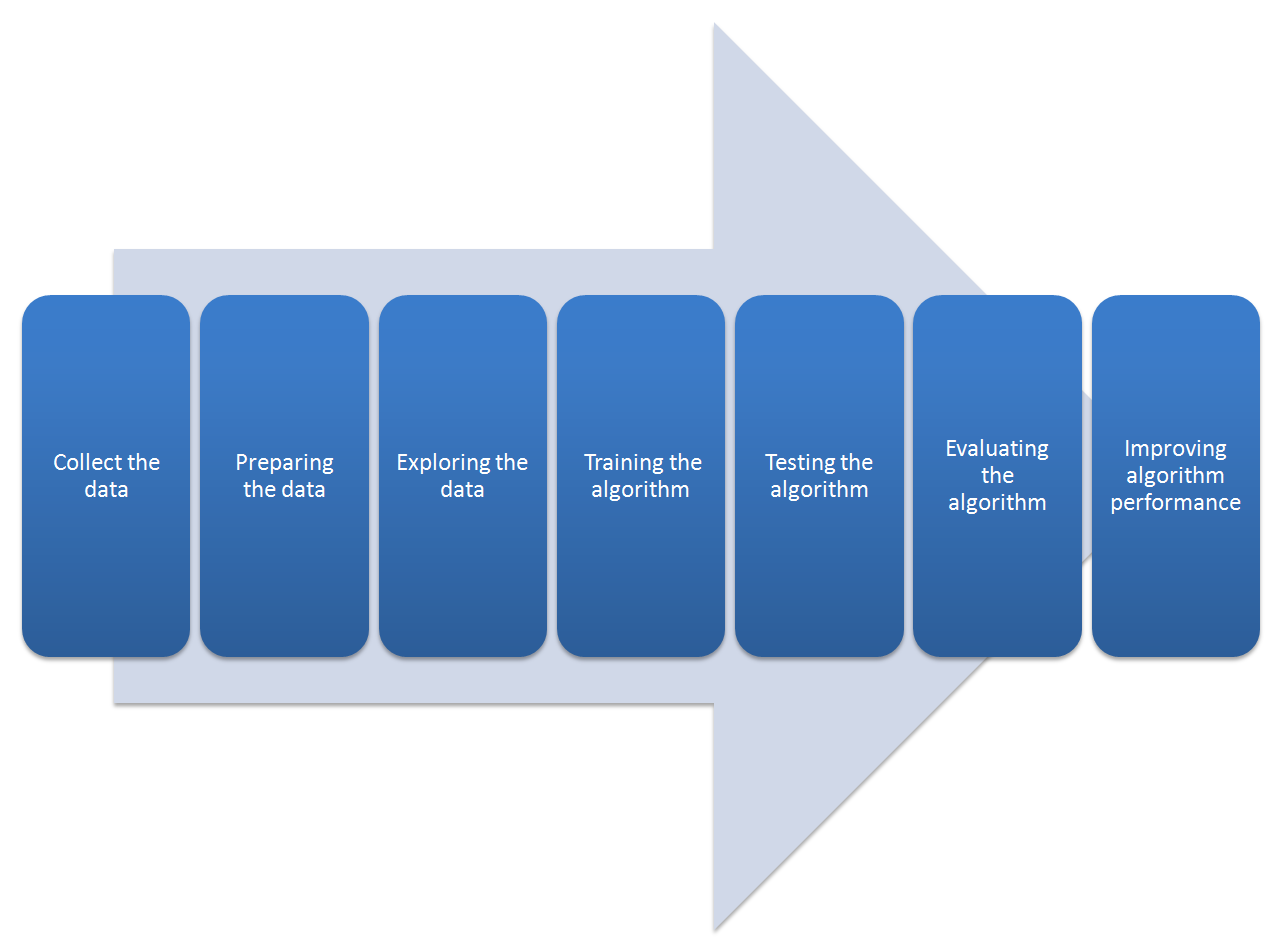1. Preparing the data: We have collected the data; now we have to prepare it for the next step. Once you have this data, you must make sure it is in a format usable by the algorithm you want to use. To do this, you may need to do some formatting. Recall that some algorithms need data in an integer format, whereas some require it in the form of strings, and finally others need it to be in a special format. We will get to this later, but the specific formatting is usually simple compared to data collection.
1. Exploring the data: At this point, we can look at the data to verify that it is actually working and we do not have a bunch of empty values. In this step, through the use of plots, we can recognize any patterns or check whether there are some data points that are vastly different from the rest of the set. Plotting data in one, two, or three dimensions can also help.
2. Training the algorithm: At this stage, it starts to get serious. The regression algorithm begins to work with the definition of the model and the next training step. The model starts to extract knowledge from the large amounts of data that we have available.
3. Testing the algorithm: In this step, we use the information learned in the previous step to see whether the model actually works. The evaluation of an algorithm is for seeing how well the model approximates the real system. In the case of regression techniques, we have some known values that we can use to evaluate the algorithm. So, if we are not satisfied, we can return to the previous steps, change some things, and retry the test.
4. Evaluating the algorithm: We have reached the point where we can apply what has been done so far. We can assess the approximation ability of the model by applying it to real data. The model, preventively trained and tested, is then valued in this phase.
5. Improving algorithm performance: Finally, we can focus on finishing the work. We have verified that the model works, we have evaluated the performance, and now we are ready to analyze it completely to identify possible room for improvement.

The generalization ability of the regression model is crucial for all other machine learning algorithms as well. Regression algorithms must not only detect the relationships between the target function and attribute values ​​in the training set, but also generalize them so that they may be used to predict new data.

It should be emphasized that the learning process must be able to capture the underlying regimes from the training set and not the specific details. Once the learning process is completed through training, the effectiveness of the model is tested further on a dataset named`testset`.

## Regression versus correlation

At the beginning of the chapter, we said that regression analysis is a statistical process for studying the relationship between variables. When considering two or more variables, one can examine the type and intensity of the relationships that exist between them. In the case where two quantitative variables are simultaneously detected for each individual, it is possible to check whether they vary simultaneously and what mathematical relationship exists between them.

The study of such relationships can be conducted through two types of analysis: regression analysis and correlation analysis. Let's understand the differences between these two types of analysis.

Regression analysis develops a statistical model that can be used to predict the values of a variable, called dependent, or more rarely predict and identify the effect based on the values of the other variable, called independent or explanatory, identified as the cause.

With correlation analysis, we can measure the intensity of the association between two quantitative variables that are usually not directly linked to the cause-effect and easily mediated by at least one third variable, but that vary jointly. In the following figure, the meanings of two analyses are shown: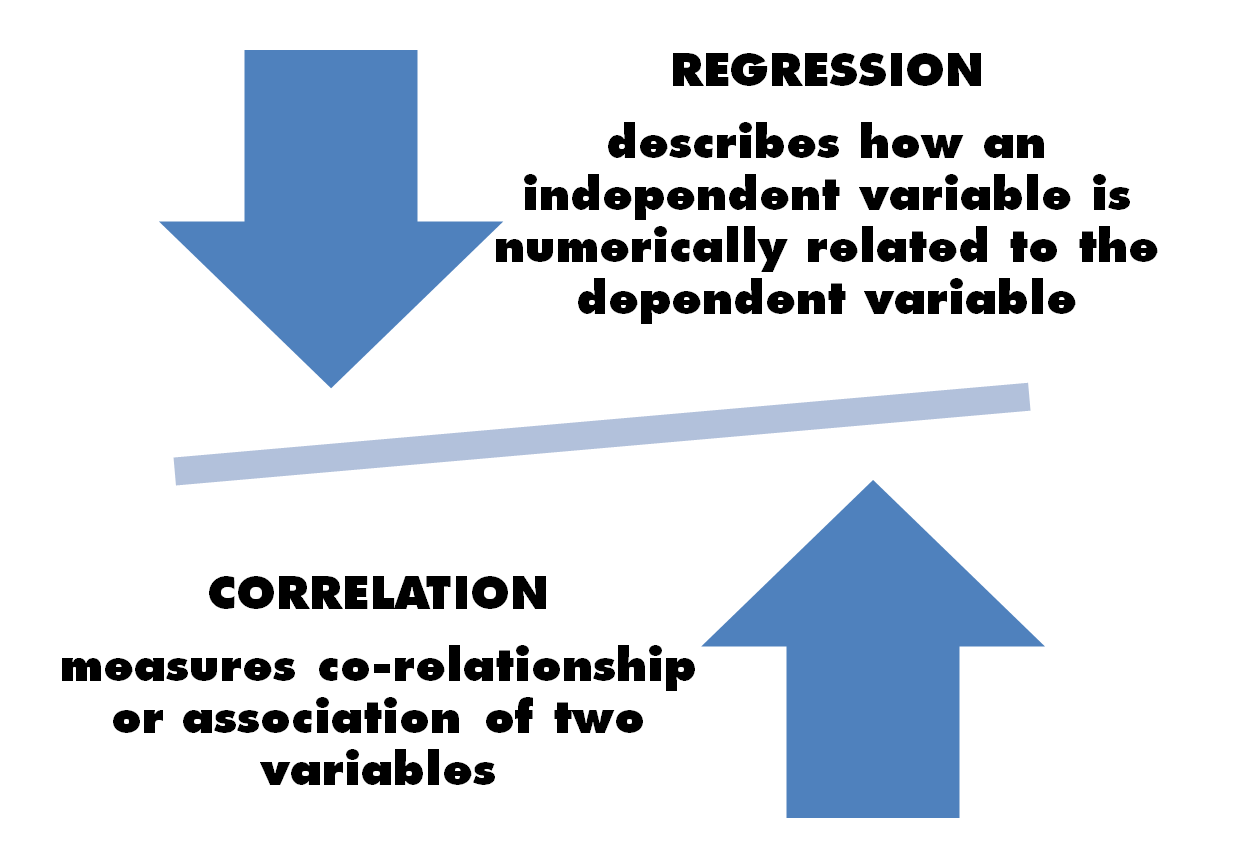In many cases, there are variables that tend to covariate, but what more can be said about this? If there is a direct dependency relation between two variables—such as whether a variable (dependent) can be determined as a function of a second variable (independent)—then a regression analysis can be used to understand the degree of the relation. For example, blood pressure depends on the subject's age. The existing data used in regression and mathematical equations is defined. Using these equations, the value of one variable can be predicted for the value of one or more variables. This equation can therefore also be used to extract knowledge from the existing data and to predict the outcomes of observations that have not been seen or tested before.

Conversely, if there is no direct dependency relation between variables—such as none of the two variables causing direct variations in the other—the covariate tendency is measured in correlation. For example, there is no direct relationship between the length and weight of an organism, in the sense that the weight of an organism can increase independently of its length. Correlation measures the association between two variables and it quantifies the strength of the relationship. To do this, it evaluates only the existing data.

To better understand the differences, we will analyze in detail the two examples just suggested. For the first example, we list the blood pressure of subjects of different ages, as shown in the following table:

 Age Blood pressure `18` `117` `22` `120` `27` `122` `32` `123` `37` `124` `42` `125` `47` `127` `52` `131` `55` `133` `62` `134` `68` `135`

A suitable graphical representation called a scatter plot can be used to depict the relationships between quantitative variables detected on the same units. In the following chapters, we will see in practice how to plot a scatter plot, so let's just make sense of the meaning.

We display this data on a scatter plot, as shown in the following figure: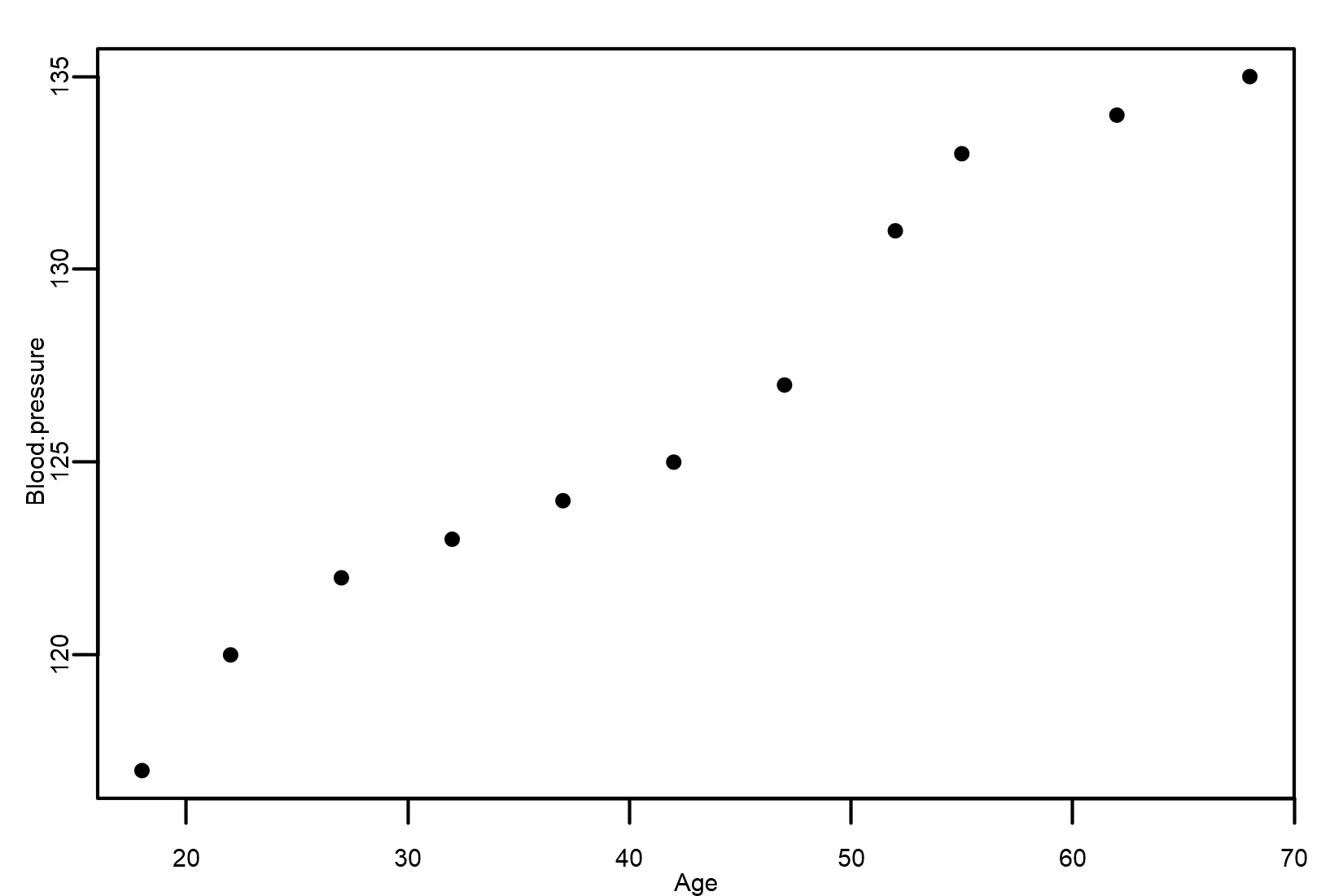By analyzing the scatter plot, we can answer the following:

• Is there a relationship that can be described by a straight line?
• Is there a relationship that is not linear?
• If the scatter plot of the variables looks similar to a cloud, it indicates that no relationship exists between both variables and one would stop at this point

The scatter plot shows a strong, positive, and linear association between Age and Blood pressure. In fact, as age increases, blood pressure increases.

Let's now look at what happens in the other example. As we have anticipated, there is no direct relationship between the length and weight of an organism, in the sense that the weight of an organism can increase independently of its length.

We can see an example of an organism's population here: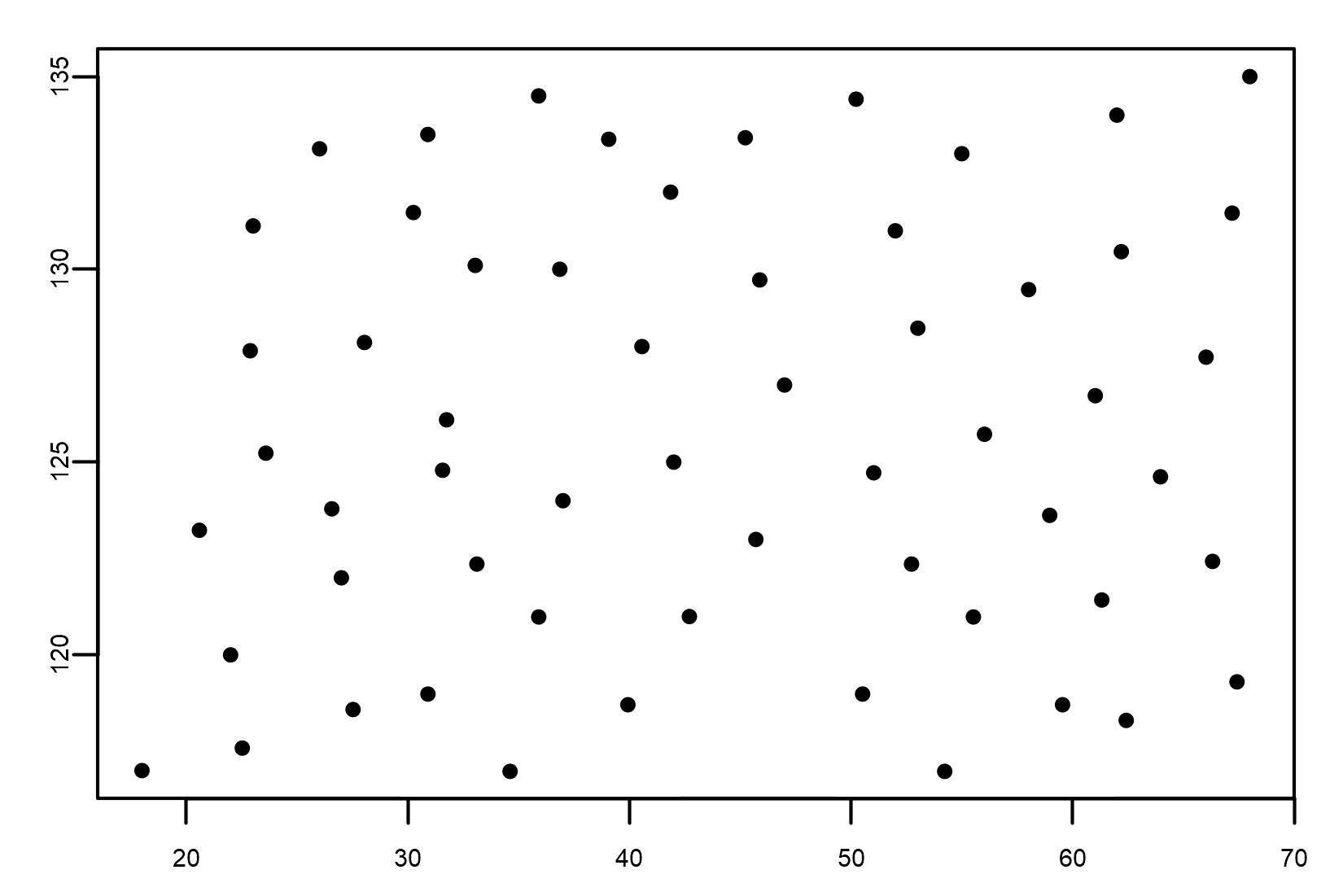Weight is not related to length; this means that there is no correlation between the two variables, and we conclude that length is responsible for none of the changes in weight.

Correlation provides a numerical measure of the relationship between two continuous variables. The resulting correlation coefficient does not depend on the units of the variables and can therefore be used to compare any two variables regardless of their units.

## Discovering different types of regression

As mentioned before, regression analysis is a statistical process for studying the relationship between a set of independent variables (explanatory variables) and the dependent variable (response variable). Through this technique, it will be possible to  understand how the value of the response variable changes when the explanatory variable is varied.

The power of regression techniques is due to the quality of their algorithms, which have been improved and updated over the years. These are divided into several main types, depending on the nature of the dependent and independent variables used or the shape of the regression line.

The reason for such a wide range of regression techniques is the variety of cases to be analyzed. Each case is based on data with specific characteristics, and each analysis is characterized by specific objectives. These specifications require the use of different types of regression techniques to obtain the best results.

How do we distinguish between different types of regression techniques? Previously, we said that a first distinction can be made based on the form of the regression line. Based on this feature, the regression analysis is divided into linear regression and nonlinear regression, as shown in the following figure (linear regression to the left and nonlinear quadratic regression to the right):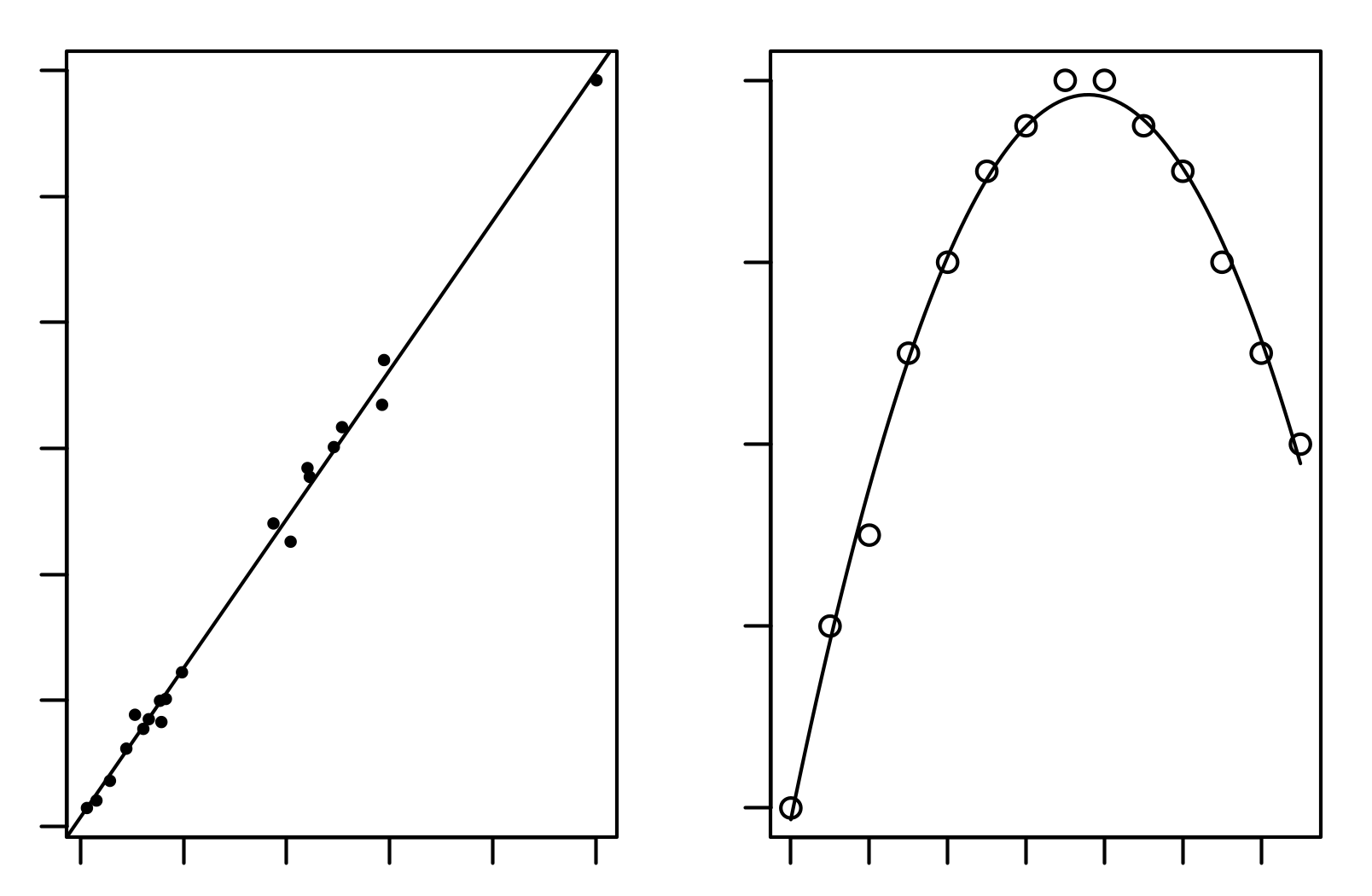It's clear that the shape of the regression line is dependent on the distribution of data. There are cases where a straight line is the regression line that best approximates the data, while in other cases, you need to fall into a curve to get the best approximation. That said, it is easy to understand that a visual analysis of the distribution of data we are going to analyze is a good practice to be done in advance. By summarizing the shape of distribution, we can distinguish the type of regression between the following:

• Linear regression
• Nonlinear regression

Let us now analyze the nature of the variables involved. In this regard, a question arises spontaneously: can the number of explanatory variables affect the choice of regression technique? The answer to this question is surely positive. For example, in the case of linear regression, if there is only one input variable, then we will do simple linear regression. If, instead, the input variables are two or more, we will need to perform multiple linear regression.

By summarizing, a simple linear regression shows the relationship between a dependent variable Y and an independent variable X. A multiple regression model shows the relationship between a dependent variable Y and multiple independent variables X. In the following figure, the types of regression imposed from the Number of the explanatory variables are shown: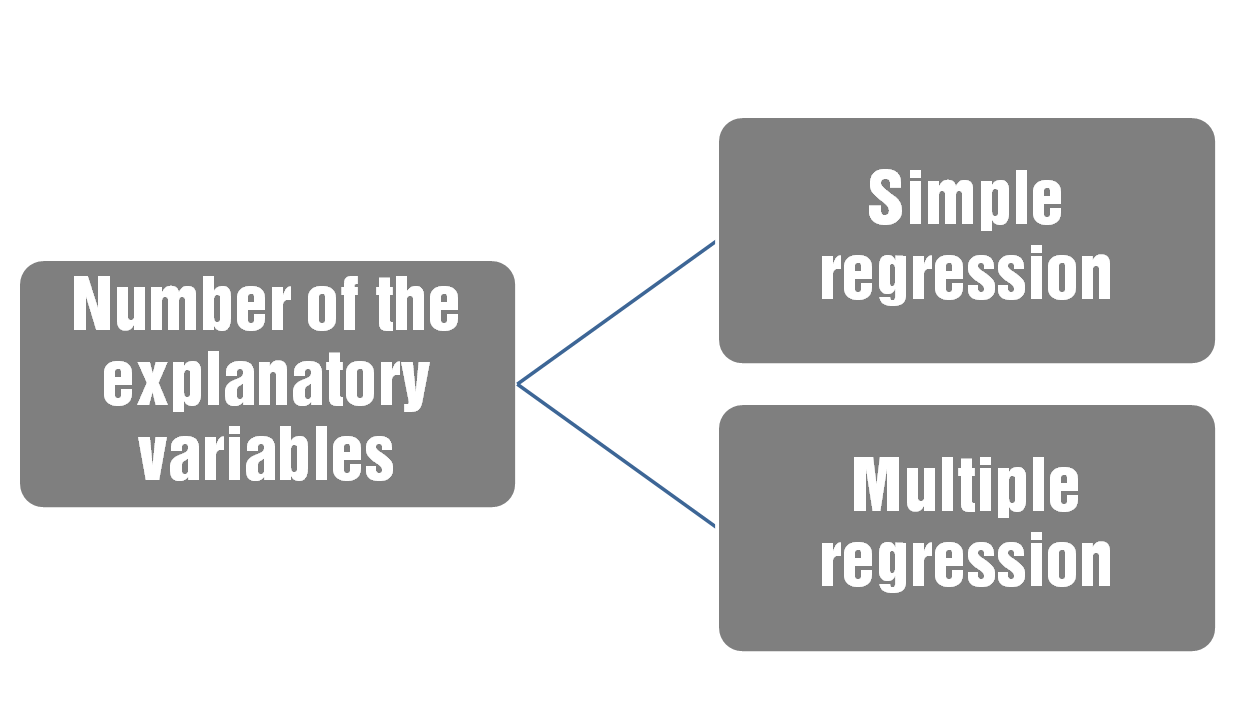What if we have multiple response variables rather than explanatory variables? In that case, we move from univariate models to multivariate models. As suggested by the name itself, multivariate regression is a technique with the help of which a single regression model can be estimated with more than one response variable. When there is more than one explanatory variable in a multivariate regression model, the model is a multivariate multiple regression.

Finally, let's see what happens when we analyze the type of variables. Usually, regression analysis is used when you want to predict a continuous response variable from a number of explanatory variables, also continuous. But this is not a limitation of regression, in the sense that such analysis is also applicable when categorical variables are at stake.

In the case of a dichotomous explanatory variable (which takes a value of zero or one), the solution is immediate. There are already two numbers (zero and one) associated to this variable, so the regression is immediately applicable. Categorical explanatory variables with more than two values can also be used in regression analyses; however, before they can be used, they need to be converted into variables that have only two levels (such as zero and one). This is called dummy coding or indicator variables.

Logistic regression should be used if the response variable is dichotomous.

## The R environment

After introducing the main topic of the book, it is time to discover the programming environment we will use for our regression analysis. As specified by the title of the book, we will perform our examples in the R environment.

R is an interpreted programming language that allows the use of common facilities for controlling the flow of information, and modular programming using a large number of functions. Most of the available features are written in R. It is also possible for the user to interface with procedures written in C, C ++, or FORTRAN.

R is a GNU project that draws its inspiration from the S language; in fact R is similar to S and can be considered as a different implementation of S. R was developed by John Chambers and his colleagues at Bell Laboratories. There are some significant differences between R and S, but a large amount of the code written for S runs unaltered under R too. R is available as free software under the terms of the Free Software Foundation's (FSF) GNU General Public License (GPL) in source code form.

Let's specify the definition we just introduced to present R. In fact, R represents a programming environment originally developed for statistical computation and for producing quality graphs. It consists of a language and runtime environment with a graphical interface, a debugger, and access to some system features. It provides the ability to run programs stored in script files. In the following figure, the `R version 3.4.1` interface is shown: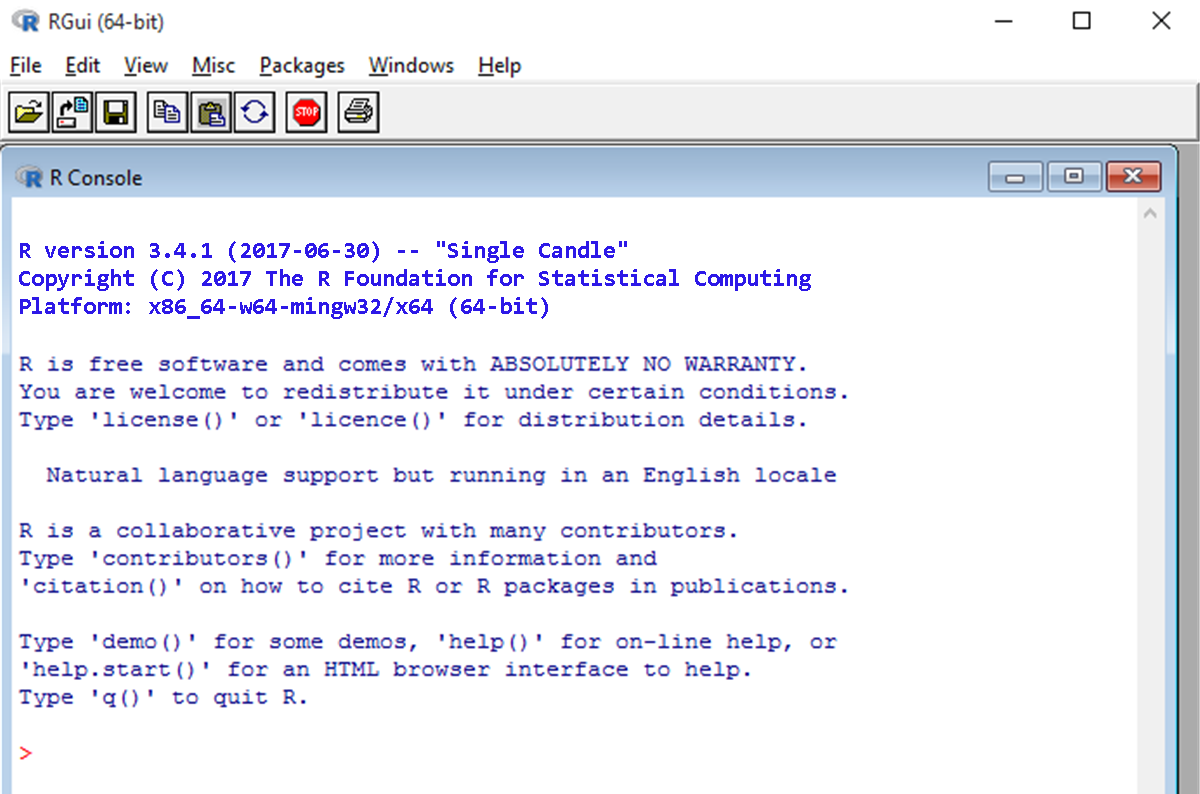R is an integrated computing system whose resources allow you to:

• Specify a set of commands and require these to run
• View results in text format
• View the charts in an auxiliary window
• Access external archives, even the web-based ones, to capture documents, data, and charts
• Permanently store results and/or graphics

What makes R so useful and explains its quick appreciation from users? The reason lies in the fact that statisticians, engineers, and scientists who have used the software over time have developed a huge collection of scripts grouped in packages. Packages written in R are able to add advanced algorithms, textured color graphics, and data mining techniques to better analyze the information contained in a database.

Its popularity among developers has resulted in its rapid rise among the most widely used programming languages, so over the last 1 year, the TIOBE index has been well ahead by seven positions. The TIOBE programming community index is a way to measure how popular a programming language is, and is updated once a month.

### Note

The TIOBE programming community index is available at the following link: https://www.tiobe.com/tiobe-index/.

These ratings are derived on the basis of the number of skilled engineers worldwide, courses, and third-party vendors, and are calculated using popular search engines such as Google, Bing, Yahoo!, Wikipedia, Amazon, YouTube, and Baidu. In the following figure, the first fifteen positions of the TIOBE index for January, 2018 are shown: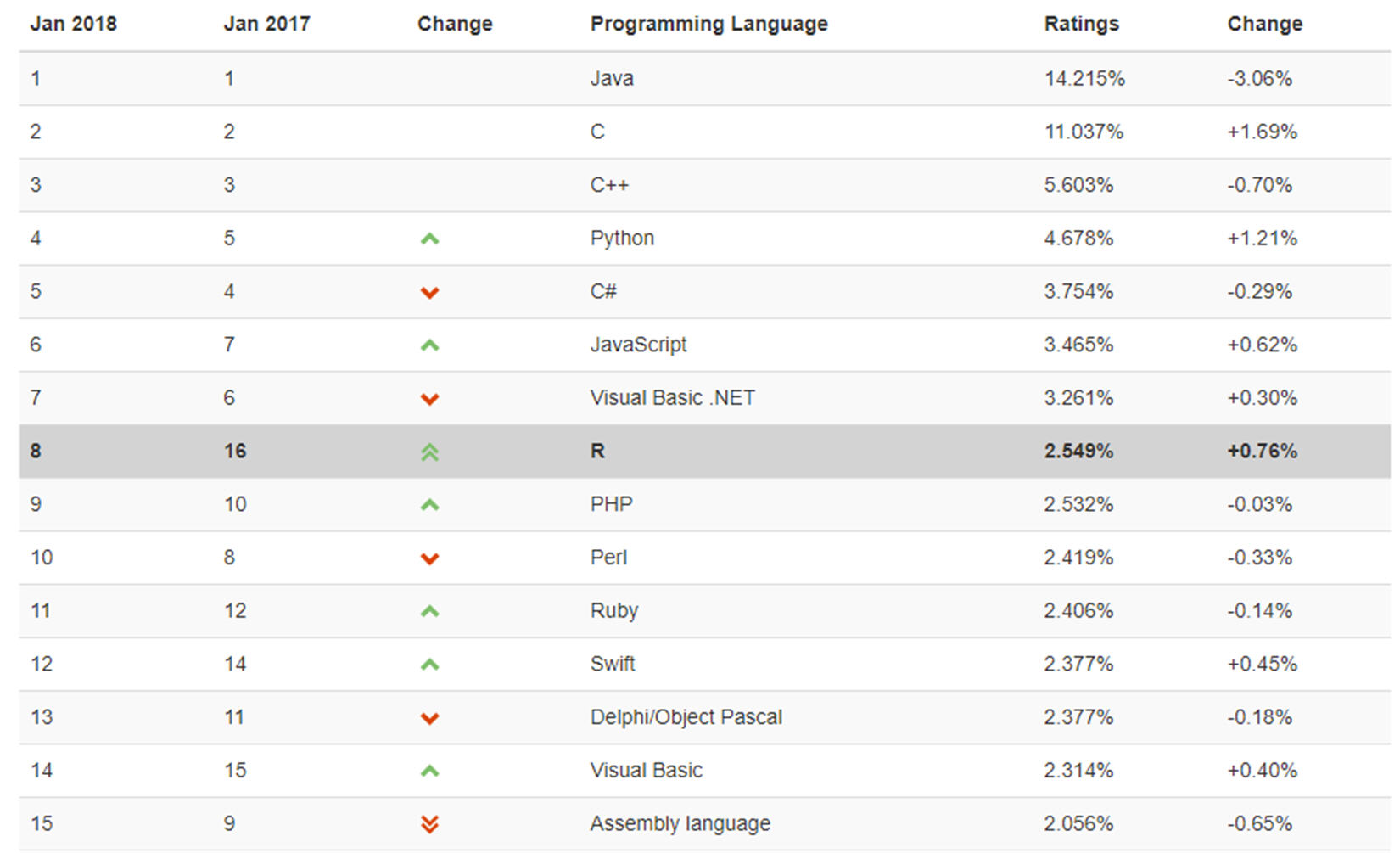R is an open source program, and its popularity reflects a change in the type of software used within the company. We remind you that open source software is free from any constraints not only on its use, but more importantly, on its development. Large IT companies such as IBM, Hewlett-Packard, and Dell are able to earn billions of dollars a year by selling servers running the Linux open source operating system, which is Microsoft Windows' competitor.

The widespread use of open source software is now a consolidated reality. Most websites are managed through an open source web application called Apache, and companies are increasingly relying on the MySQL open source database to store information. Finally, many people see the end results of this technology using the open source Firefox web browser.

R is in many respects similar to other programming languages, such as C, Java, and Perl. Because of its features, it is extremely simple and fast to do a wide range of computing tasks, as it provides quick access through various commands. For statisticians, however, R is particularly useful because it contains a number of integrated mechanisms (built-in functions) to organize data, to perform calculations on information, and to create graphic representations of databases.

Indeed, R is highly extensible and provides a wide variety of statistical (linear and nonlinear modeling, classical statistical tests, time-series analysis, classification, clustering, and so on) and graphical techniques. There is also a wide range of features that provide a flexible graphical environment for creating various types of data presentations. Additional forms of (add-on) packages are available for a variety of specific purposes. These are topics that we will look at in the following sections.

## Installing R

After that detailed description of the programming environment R, it is time to install it on our machine. To do this, we will have to get the installation package first.

### Note

The packages we will need to install are available on the official website of the language, Comprehensive R Archive Network (CRAN), at the following URL: https://www.r-project.org/.

CRAN is a network of File Transfer Protocol (FTP) and web servers located around the world that stores identical source and documentation versions of R. CRAN is directly accessible from R's site, and on this site you can also find information about R, some technical manuals, the R magazine, and details about R-developed packages that are stored in CRAN repositories.

Of course, before you download the software versions, we will have to inform you of the type of machine you need and the operating system that must be installed on it. Remember, however, that R is practically available for all operating systems in circulation. In the following screenshot, the CRAN web page is shown: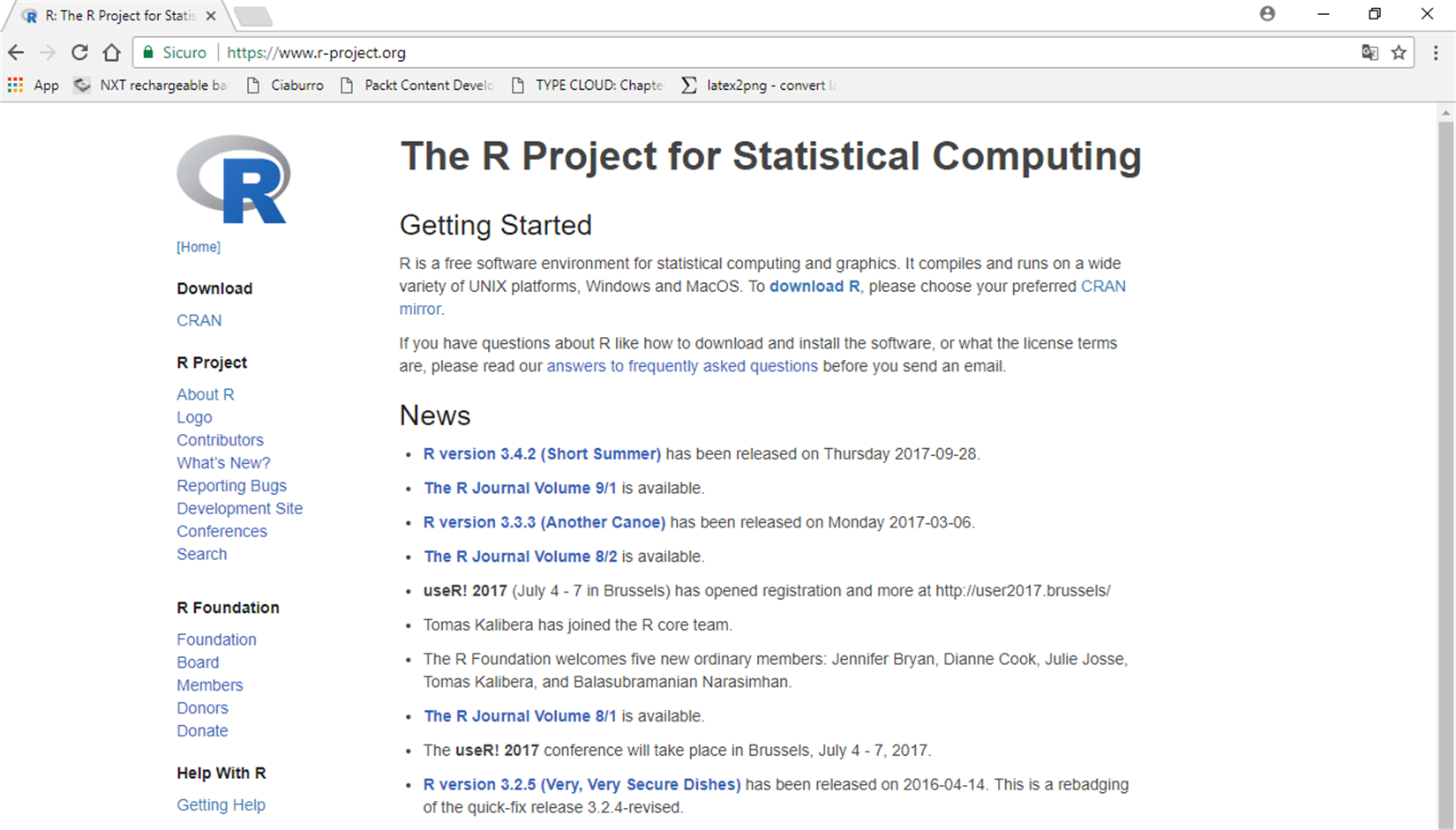In the drafting period of this book, the current version of the environment R is 3.4.1, which represents the stable one, and that is why, in the examples that will accompany us in the subsequent sections, we will refer to that version.

The following list shows the OSs supported:

• Windows
• macOS
• Unix

In computer science, installation is the procedure whereby the software is copied and configured on the machine. Generally, the software is distributed as a compressed file package, which includes an interface that facilitates and automates the installation (installer).

The installation creates folders on the disk, where all the files used for the program configuration are contained, and the links to make it easier to execute and write the necessary configuration parameters. In the following screenshot, we can see CRAN with all the tools needed for proper software installation: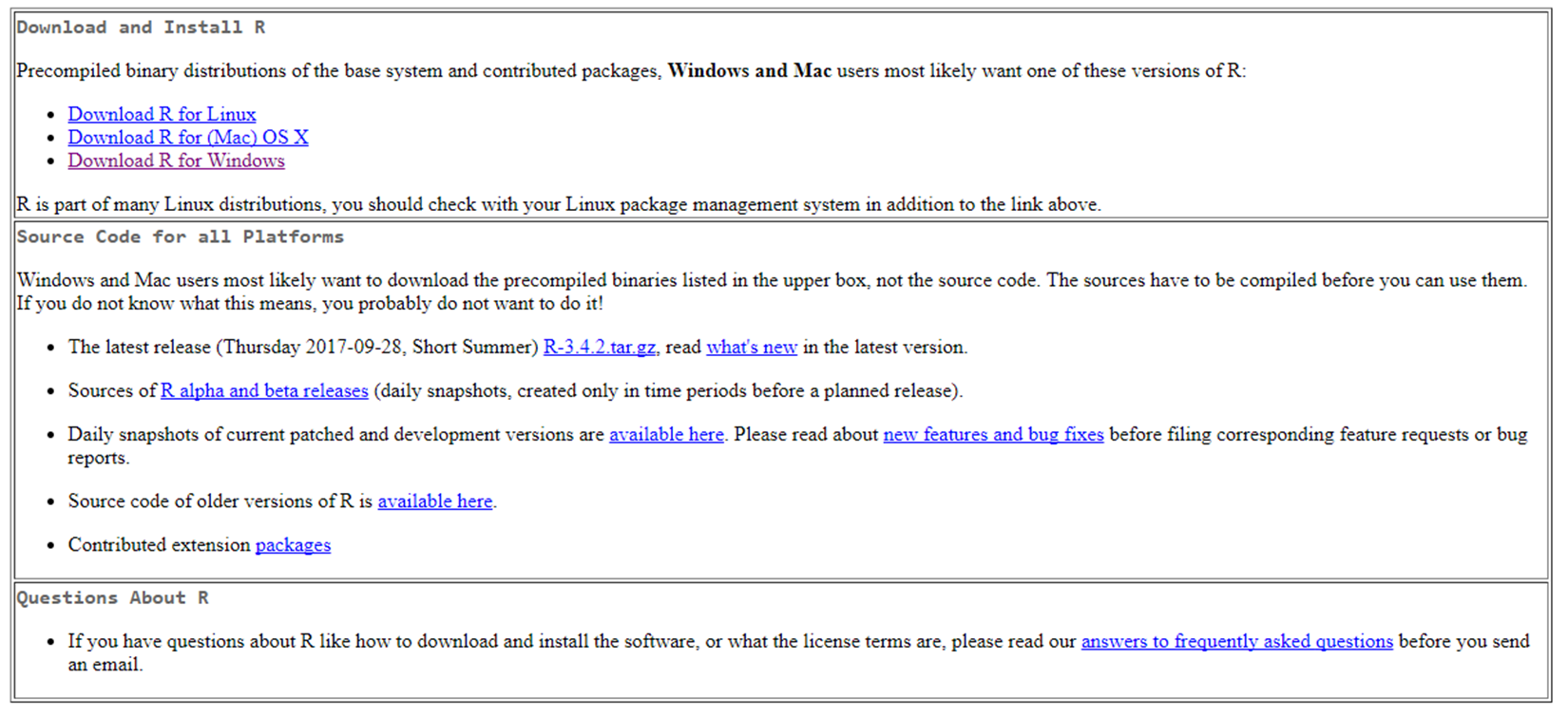There are essentially two ways to install R:

• Using existing distributions in the form of binaries
• Using source code

### Using precompiled binary distribution

Binary distribution is the simplest choice; it works on most machines and will be the one we will use to make the job as simple as possible. This is a compiled version of R which can be downloaded and installed directly on our system.

#### Installing on Windows

For the Windows operating system, this version looks like a single EXE file (downloadable from the CRAN site), which can be easily installed with a double-click on it and by following the few steps of the installation. These are the automated installation procedures, the so-called installers, through which the installation phase of the software is reduced by the user to the need to have clicked on the buttons a number of times. Once the process is completed, you can start using R via the icon that will appear on the desktop or through the link available in the list of programs that can be used in our system.

#### Installing on macOS

Similarly, for macOS, R is available with a unique installation file with a PKG extension; it can be downloaded and installed on our system. The following screenshot shows the directory containing binaries for a base distribution and packages to run on macOS X (release 10.6 and later) extracted from the CRAN website: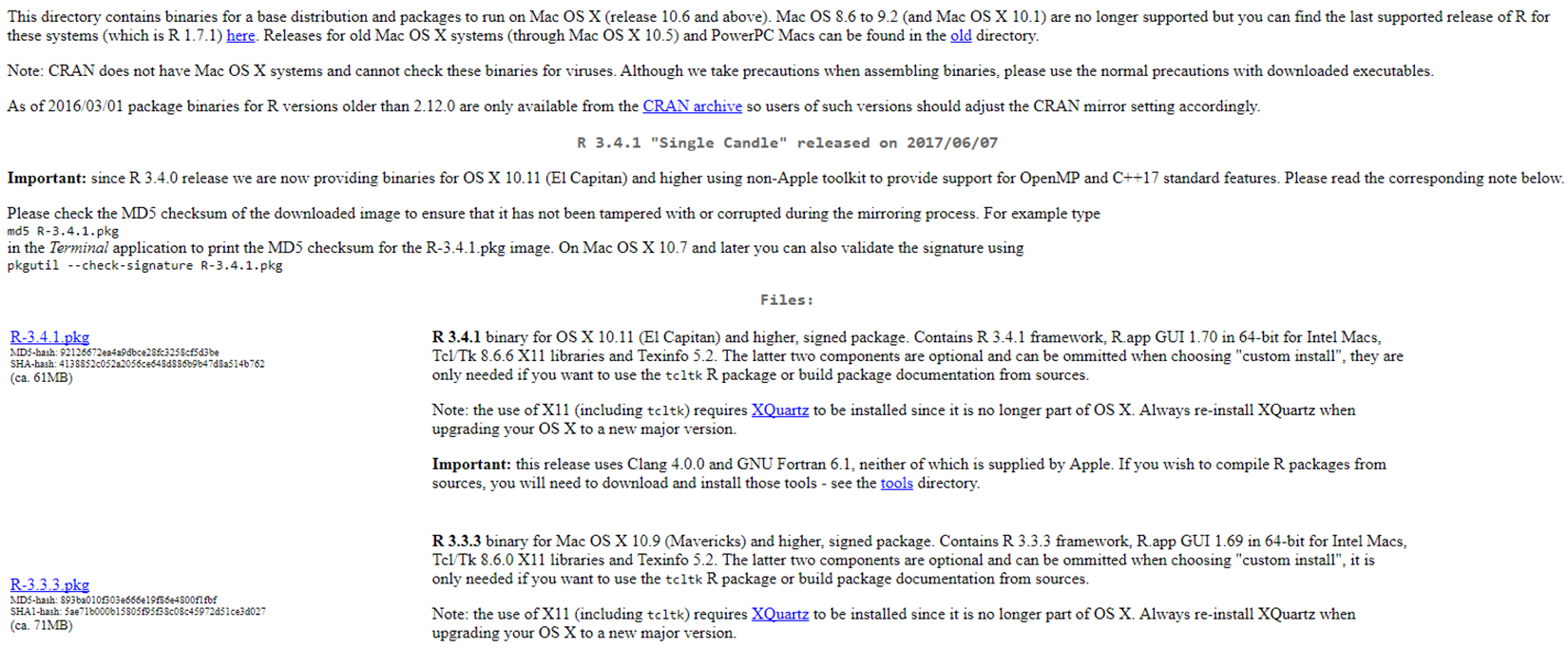#### Installing on Linux

For a Linux system, there are several versions of the installation file. In the download section, you must select the appropriate version of R, according to the Linux distribution installed on your machine. Installation packages are available in two main formats, `.rpm` file for Fedora, SUSE, and Mandriva, and `.deb` extensions for Ubuntu, Debian, and Linux Mint.

### Installation from source code

R's installation from source code is available for all supported platforms, though it is not as easy to perform compared to the binary distribution we've just seen. It is especially hard on Windows, since the installation tools are not part of the system.

### Note

Detailed information on installation procedures from source code for Windows, and necessary tools, are available on the CRAN website, at https://cran.r-project.org/doc/manuals/r-release/R-admin.html.

On Unix-like systems, the process, on the other hand, is much simpler; the installation must be done following the usual procedure, which uses the following commands:

```./configure
make
make install```

These commands, assuming that compilers and support libraries are available, lead to the proper installation of the R environment on our system.

## RStudio

To program with R, we can use any text editor and a simple command-line interface. Both of these tools are already present on any operating system, so if you don't want to install anything more, you will be able to ignore this step.

Some find it more convenient to use an Integrated Development Environment (IDE); in this case, as there are several available, both free and paid, you'll be spoilt for choice. Having to make a choice, I prefer the RStudio environment.

RStudio is a set of integrated tools designed to help you be more productive with R. It includes a console, syntax-highlighting editor that supports direct code execution, and a variety of robust tools for plotting, viewing history, debugging, and managing your workspace.

### Note

RStudio is available at the following URL: https://www.rstudio.com/.

In the following screenshot you will see the main page of the RStudio website: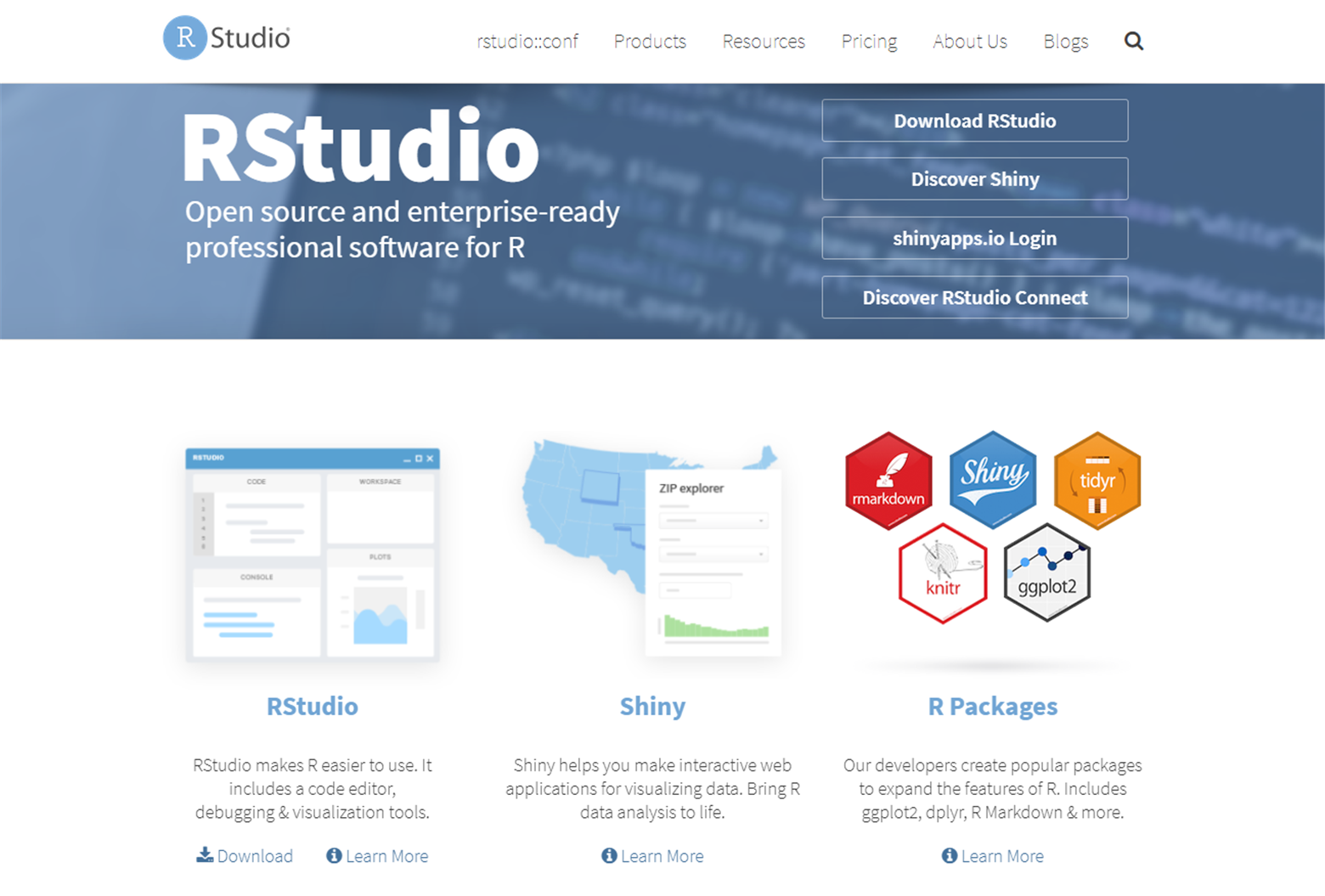This is a popular IDE available in the open source, free, and commercial versions, which works on most operating systems. RStudio is probably the only development environment developed specifically for R. It is available for all major platforms (Windows, Linux, and macOS X) and can run on a local machine such as our computer or even on the web using RStudio Server. With RStudio Server, you can provide a browser-based interface (the so-called IDE) to an R version running on a remote Linux server. It integrates several features that are really useful, especially if you use R for more complex projects or if you want to have more than one developer on a project.

The environment is made up of four different areas:

• Scripting area: In this area we can open, create, and write our scripts
• Console area: This zone is the actual R console where commands are executed
• Workspace History area: In this area you can find a list of all the objects created in the workspace where we are working
• Visualization area: In this area we can easily load packages and open R help files, but more importantly, we can view charts

The following screenshot shows the RStudio environment:With the use of RStudio, our work will be considerably simplified, displaying all the resources needed in one window.

## R packages for regression

Previously, we have mentioned the R packages, which allow us to access a series of features to solve a specific problem. In this section, we will present some packages that contain valuable resources for regression analysis. These packages will be analyzed in detail in the following chapters, where we will provide practical applications.

### The R stats package

R `stats` is a package that contains many useful functions for statistical calculations and random number generation. In the following table you will see some of the information on this package:

 Package `stats` Date October 3, 2017 Version 3.5.0 Title The R stats package Author R core team and contributors worldwide

There are so many functions in the package; we will only mention the ones that are closest to regression analysis. These are the most useful functions used in regression analysis:

• `lm`: This function is used to fit linear models. It can be used to carry out regression, single stratum analysis of variance, and analysis of co-variance.
• `summary.lm`: This function returns a summary for linear model fits.
• `coef`: With the help of this function, coefficients from objects returned by modeling functions can be extracted. Coefficients is an alias for it.
• `fitted`: Fitted values are extracted by this function from objects returned by modeling functions fitted. Values are an alias for it.
• `formula`: This function provides a way of extracting formulae which have been included in other objects.
• `predict`: This function predicts values based on linear model objects.
• `residuals`: This function extracts model residuals from objects returned by modeling functions.
• `confint`: This function computes confidence intervals for one or more parameters in a fitted model. Base has a method for objects inheriting from the `lm` class.
• `deviance`: This function returns the deviance of a fitted model object.
• `influence.measures`: This suite of functions can be used to compute some of the regression (leave-one-out deletion) diagnostics for linear and generalized linear models (GLM).
• `lm.influence`: This function provides the basic quantities used when forming a wide variety of diagnostics for checking the quality of regression fits.
• `ls.diag`: This function computes basic statistics, including standard errors, t-values, and p-values for the regression coefficients.
• `glm`: This function is used to fit GLMs, specified by giving a symbolic description of the linear predictor and a description of the error distribution.
• `loess`: This function fits a polynomial surface determined by one or more numerical predictors, using local fitting.
• `loess.control`: This function sets control parameters for `loess` fits.
• `predict.loess`: This function extracts predictions from a `loess` fit, optionally with standard errors.
• `scatter.smooth`: This function plots and adds a smooth curve computed by `loess` to a scatter plot.

What we have analyzed are just some of the many functions contained in the stats package. As we can see, with the resources offered by this package we can build a linear regression model, as well as GLMs (such as multiple linear regression, polynomial regression, and logistic regression). We will also be able to make model diagnosis in order to verify the plausibility of the classic hypotheses underlying the regression model, but we can also address local regression models with a non-parametric approach that suits multiple regressions in the local neighborhood.

### The car package

This package includes many functions for: ANOVA analysis, matrix and vector transformations, printing readable tables of coefficients from several regression models, creating residual plots, tests for the autocorrelation of error terms, and many other general interest statistical and graphing functions.

In the following table you will see some of the information on this package:

 Package `car` Date June 25, 2017 Version 2.1-5 Title Companion to Applied Regression Author John Fox, Sanford Weisberg, and many others

The following are the most useful functions used in regression analysis contained in this package:

• `Anova`: This function returns ANOVA tables for linear and GLMs
• `linear.hypothesis`: This function is used for testing a linear hypothesis and methods for linear models, GLMs, multivariate linear models, and linear and generalized linear mixed-effects models
• `cookd`: This function returns Cook's distances for linear and GLMs
• `outlier.test`: This function reports the Bonferroni p-values for studentized residuals in linear and GLMs, based on a t-test for linear models and a normal-distribution test for GLMs
• `durbin.watson`: This function computes residual autocorrelations and generalized Durbin-Watson statistics and their bootstrapped p-values
• `levene.test`: This function computes Levene's test for the homogeneity of variance across groups
• `ncv.test`: This function computes a score test of the hypothesis of constant error variance against the alternative that the error variance changes with the level of the response (fitted values), or with a linear combination of predictors

What we have listed are just some of the many functions contained in the `stats` package. In this package, there are also many functions that allow us to draw explanatory graphs from information extracted from regression models as well as a series of functions that allow us to make variables transformations.

### The MASS package

This package includes many useful functions and data examples, including functions for estimating linear models through generalized least squares (GLS), fitting negative binomial linear models, the robust fitting of linear models, and Kruskal's non-metric multidimensional scaling.

In the following table you will see some of the information on this package:

 Package `MASS` Date October 2, 2017 Version 7.3-47 Title Support Functions and Datasets for Venables and Ripley's MASS Author Brian Ripley, Bill Venables, and many others

The following are the most useful functions used in regression analysis contained in this package:

• `lm.gls`: This function fits linear models by GLS
• `lm.ridge`: This function fist a linear model by Ridge regression
• `glm.nb`: This function contains a modification of the system function
• `glm()`: It includes an estimation of the additional parameter, theta, to give a negative binomial GLM
• `polr`: A logistic or probit regression model to an ordered factor response is fitted by this function
• `lqs`: This function fits a regression to the good points in the dataset, thereby achieving a regression estimator with a high breakdown point
• `rlm`: This function fits a linear model by robust regression using an M-estimator
• `glmmPQL`: This function fits a GLMM model with multivariate normal random effects, using penalized quasi-likelihood (PQL
• `boxcox`: This function computes and optionally plots profile log-likelihoods for the parameter of the Box-Cox power transformation for linear models

As we have seen, this package contains many useful features in regression analysis; in addition there are numerous datasets that we can use for our examples that we will encounter in the following chapters.

### The caret package

This package contains many functions to streamline the model training process for complex regression and classification problems. The package utilizes a number of R packages.

In the following table you will see listed some of the information on this package:

 Package `caret` Date September 7, 2017 Version 6.0-77 Title Classification and Regression Training Author Max Kuhn and many others

The most useful functions used in regression analysis in this package are as follows:

• `train`: Predictive models over different tuning parameters are fitted by this function. It fits each model, sets up a grid of tuning parameters for a number of classification and regression routines, and calculates a resampling-based performance measure.
• `trainControl`: This function permits the estimation of parameter coefficients with the help of resampling methods like cross-validation.
• `varImp`: This function calculates variable importance for the objects produced by train and method-specific methods.
• `defaultSummary`: This function calculates performance across resamples. Given two numeric vectors of data, the mean squared error and R-squared error are calculated. For two factors, the overall agreement rate and Kappa are determined.
• `knnreg`: This function performs K-Nearest Neighbor (KNN) regression that can return the average value for the neighbors.
• `plotObsVsPred`: This function plots observed versus predicted results in regression and classification models.
• `predict.knnreg`: This function extracts predictions from the KNN regression model.

The `caret` package contains hundreds of machine learning algorithms (also for regression), and renders useful and convenient methods for data visualization, data resampling, model tuning, and model comparison, among other features.

### The glmnet package

This package contains many extremely efficient procedures in order to fit the entire Lasso or ElasticNet regularization path for linear regression, logistic and multinomial regression models, Poisson regression, and the Cox model. Multiple response Gaussian and grouped multinomial regression are the two recent additions.

In the following table you will see listed some of the information on this package:

 Package `glmnet` Date September 21, 2017 Version 2.0-13 Title Lasso and Elastic-Net Regularized Generalized Linear Models Author Jerome Friedman, Trevor Hastie, Noah Simon, Junyang Qian, and Rob Tibshirani

The following are the most useful functions used in regression analysis contained in this package:

• `glmnet`:  A GLM is fit by this function via penalized maximum likelihood. The regularization path is computed for the Lasso or ElasticNet penalty at a grid of values for the regularization parameter lambda. This function can also deal with all shapes of data, including very large sparse data matrices. Finally, it fits linear, logistic and multinomial, Poisson, and Cox regression models.
• `glmnet.control`: This function views and/or changes the factory default parameters in `glmnet`.
• `predict.glmnet`: This function predicts fitted values, logits, coefficients, and more from a fitted `glmnet` object.
• `print.glmnet`: This function prints a summary of the `glmnet` path at each step along the path.
• `plot.glmnet`: This function produces a coefficient profile plot of the coefficient paths for a fitted `glmnet` object.
• `deviance.glmnet`: This function computes the deviance sequence from the `glmnet` object.

As we have mentioned, this package fits Lasso and ElasticNet model paths for regression, logistic, and multinomial regression using coordinate descent. The algorithm is extremely fast, and exploits sparsity in the input matrix where it exists. A variety of predictions can be made from the fitted models.

### The sgd package

This package contains a fast and flexible set of tools for large scale estimation. It features many stochastic gradient methods, built-in models, visualization tools, automated hyperparameter tuning, model checking, interval estimation, and convergence diagnostics.

In the following table you will see listed some of the information on this package:

 Package `sgd` Date January 5, 2016 Version 1.1 Title Stochastic Gradient Descent for Scalable Estimation Author Dustin Tran, Panos Toulis, Tian Lian, Ye Kuang, and Edoardo Airoldi

The following are the most useful functions used in regression analysis contained in this package:

• `sgd`: This function runs Stochastic Gradient Descent (SGD) in order to optimize the induced loss function given a model and data
• `print.sgd`: This function prints objects of the `sgd` class
• `predict.sgd`: This function forms predictions using the estimated model parameters from SGD
• `plot.sgd`: This function `plots` objects of the `sgd` class

### The BLR package

This package performs a special case of linear regression named Bayesian linear regression. In Bayesian linear regression, the statistical analysis is undertaken within the context of a Bayesian inference.

In the following table you will see listed some of the information on this package:

 Package `BLR` Date December 3, 2014 Version 1.4 Title Bayesian Linear Regression Author Gustavo de los Campos, Paulino Perez Rodriguez

The following are the most useful functions used in regression analysis contained in this package:

• `BLR`: This function was designed to fit parametric regression models using different types of shrinkage methods.
• `sets`: This is a vector (599x1) that assigns observations to ten disjointed sets; the assignment was generated at random. This is used later to conduct a 10-fold CV.

### The Lars package

This package contains efficient procedures for fitting an entire Lasso sequence with the cost of a single least squares fit. Least angle regression and infinitesimal forward stagewise regression are related to the Lasso.

In the following table you will see listed some of the information on this package:

 Package `Lars` Date April 23, 2013 Version 1.2 Title Least Angle Regression, Lasso and Forward Stagewise Author Trevor Hastie and Brad Efron

The following are the most useful functions used in regression analysis contained in this package:

• `lars`: This function fits least angle regression and Lasso and infinitesimal forward stagewise regression models.
• `summary.lars`: This function produces an ANOVA-type summary for a `lars` object.
• `plot.lars`: This function produce a plot of a `lars` fit. The default is a complete coefficient path.
• `predict.lars`: This function make predictions or extracts coefficients from a fitted `lars` model.

## Summary

In this chapter, we were introduced to the basic concepts of regression analysis, and then we discovered different types of statistical processes. Starting from the origin of the term regression, we explored the meaning of this type of analysis. We have therefore gone through analyzing the real cases in which it is possible to extract knowledge from the data at our disposal.

Then, we analyzed how to build regression models step by step. Each step of the workflow was analyzed to understand the meaning and the operations to be performed. Particular emphasis was devoted to the generalization ability of the regression model, which is crucial for all other machine learning algorithms.

We explored the fundamentals of regression analysis and correlation analysis, which allows us to study the relationships between variables. We understood the differences between these two types of analysis.

In addition, an introduction, background information, and basic knowledge of the R environment were covered. Finally, we explored a number of essential packages that R provides for understanding the amazing world of regression.

In the next chapter, we will approach regression with the simplest algorithm: simple linear regression. First, we will describe a regression problem in terms of where to fit a regressor and then we will provide some intuitions underneath the math formulation. Then, the reader will learn how to tune the model for high performance and deeply understand every parameter of it. Finally, some tricks will be described to lower the complexity and scale the approach.